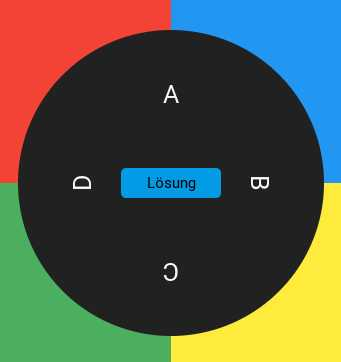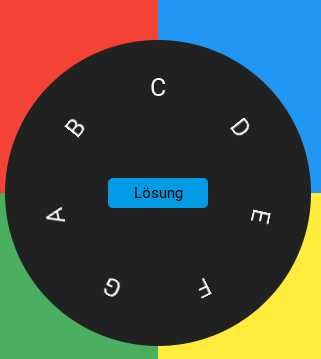# Circular/Round Text?

Hi,

is it possible to make a text circular/round? The text is an observable which is also changing.

Hi!

What do you mean by circular/round? Can you please post an image that shows what you want?

Don`t have a picture. Like a ring (or wheel) where the text goes around the wheel. E.g. the data printed on a car tire. Is this clear? Otherwise I search for an image.

There is no way to do that with one long string, but if you can split your string into multiple `Text` objects, you can use `Rotation` and `Translation` rotate them around in a circle. There is also a `CircularLayout` you can use, but I have never used it.

Ok, I will give that a try.

Hi,

I come close, but need a hint, how to align it perfectly.

``````
<App Theme="Basic" Background="#eeef">
<JavaScript>

var Observable = require("FuseJS/Observable");
var roundText = Observable();
var word = "ABCD";
var sw = word.toUpperCase().split("");
var angleDelta = Math.floor(360/(sw.length));

console.log("angleDelta:" + angleDelta);
for (var i = 0; i < sw.length; i++)
{
currentAngle =  i*angleDelta + 90;
roundText.add({ char: sw[i], angle:  currentAngle });
console.log("char: " + sw[i] + "  angle: " + currentAngle );
}
module.exports = {
roundText: roundText,
};
</JavaScript>
<ClientPanel>
<DockPanel>
<Panel>
<Circle Height="180" Width="180" Fill="#FDD835" />
<Circle Height="300" Width="300" Fill="#f44336" >
<CircleLayout StartAngleDegrees="-360" EndAngleDegrees="0"/>
<Each Items="{roundText}">
<Rectangle  Height="35" Width="35">
<Text Value="{char}" FontSize="20" Layer="Overlay"/>
<WhileActive>
<Rotate Degrees="{angle}" Duration="0"/>
</WhileActive>
</Rectangle>
</Each>
<Stroke Alignment="Center"  Width="5" Brush="#212121" />
</Circle>
</Panel>
</DockPanel>
</ClientPanel>
</App>

```

Hi,

I managed to get what I wanted with this code (extract). JS:

``````roundText.clear();
var sw = randomWord.value.toUpperCase().split("") ;
var angleDelta = 360/(sw.length);
for (var i = 0; i < sw.length; i++) {
currentAngle =  i*angleDelta ;
roundText.add({ char: sw[i], angle:  currentAngle });
}

``````

UX:

``````<Circle Height="300" Width="300" Fill="#212121" >
<CircleLayout StartAngleDegrees="270" EndAngleDegrees="630"/>
<Each Items="{roundText}">
<Rectangle  Alignment="Center" Height="60" Width="60">
<Text Value="{char}" Alignment="Center" FontSize="25" Layer="Overlay"/>
<WhileActive>
<Rotate Degrees="{angle}" Duration="0.8"/>
</WhileActive>
</Rectangle>
</Each>
</Circle>

``````

Great, thanks for sharing the codeWould love a screenshot too!

The start location of the word is random (on purpose).The longer the word the more it shifts to the outer border of the circle. Not perfect, but will do for my purpose.